From colors and fonts to grid layouts and carousels, CSS shapes how your website looks and how much your visitors will enjoy using it. That’s why it’s so important to master this language and, if you have the time, an accompanying framework like Bootstrap CSS.

Once you dig deeper into what CSS can do, however, you’ll learn that its capabilities extend beyond settings colors, fonts, and layouts. One advanced feature of CSS is transformation, allowing you to change the positions and shapes of elements on a page.

By understanding the transform property, you’ll unlock ways to further build unique and engaging interfaces from scratch. So, in this post, I’ll show you everything you need to know to start using the CSS transform property, including the many ways you can manipulate elements in two and three dimensions, and even how to animate such effects.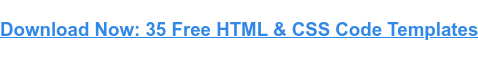A CSS transform rule is written as follows:

` `transform: value(argument);``

...where value is the type of transformation and argument is the extent to which the transformation is applied. Note that some transformation declarations can take multiple arguments.

But, this explanation doesn’t mean much without examples of the transform property in action, so let’s explore some.

## 2D CSS Transformations

CSS transformations can be split into two categories, two-dimensional and three-dimensional. We’ll look at two-dimensional transformations first. Two-dimensional CSS transformations operate on the X (horizontal) and Y (vertical) axes.

In my examples, I’ll apply the transformation effects to a basic square div element. The blue div represents the untransformed element, and the orange div shows the same element with the transformation effect applied.

### CSS Transform: Translate

The translate() method translates, or moves, a page element up, down, left, and/or right on the page by a specified amount. In the parenthesis, the first number specifies the horizontal distance, and the second number specifies the vertical distance.

For example, we can translate our div by a number of pixels:

` `transform: translate(100px, 75px);``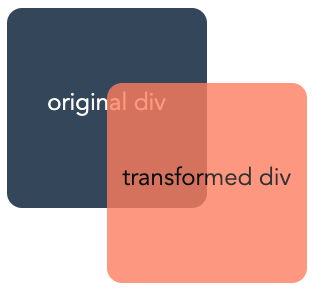You can also use percentages to specify the translation. Percentages move the element a horizontal/vertical distance proportional to its set width/height:

` `transform: translate(100%, 50%);``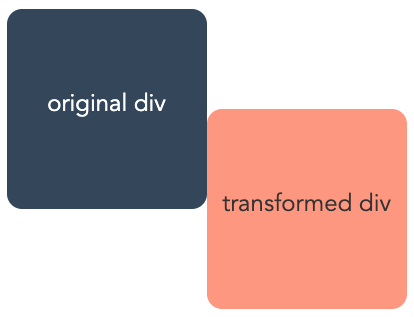In addition to translate(), we also have the translateX() and translateY() methods. translateX() moves an element only horizontally, and takes one argument:

` `transform: translateX(100px);``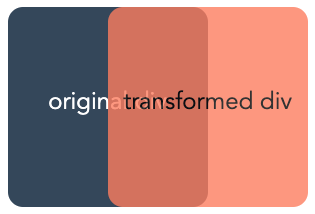Similarly, the translateY() method moves an element vertically. It also takes just one argument:

` `transform: translateY(100px);``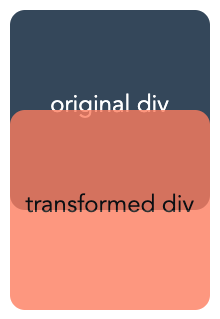### CSS Transform: Scale

The scale() method changes the size of the target element. If we provide one argument, this increases or decreases the size of our div by a multiple of its original size:

` `transform: scale(2);``` `transform: scale(0.75);``If we supply two arguments (separated by a comma), the first argument specifies the horizontal scaling and the second specifies the vertical scaling:

` `transform: scale(4, 2);``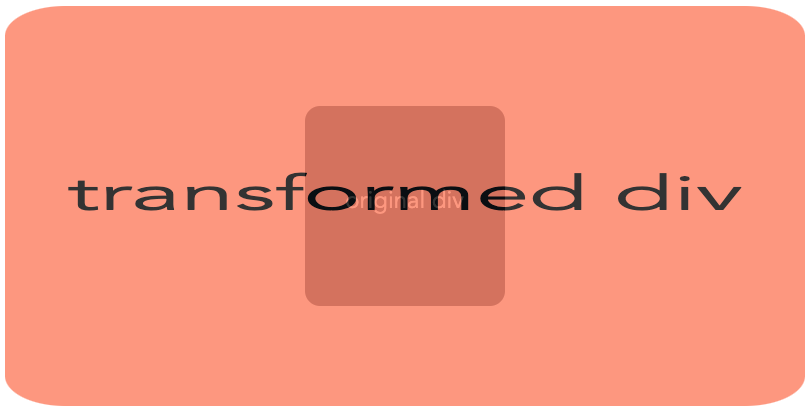The scaleX() method changes only the horizontal scaling of an element. It takes one argument:

` `transform: scaleX(2);``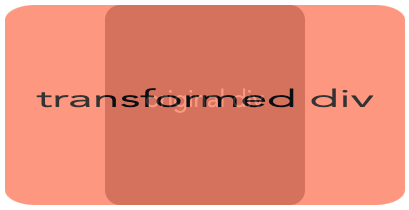scaleY() does the same as scaleX(), but vertically:

` `transform: scaleY(2);``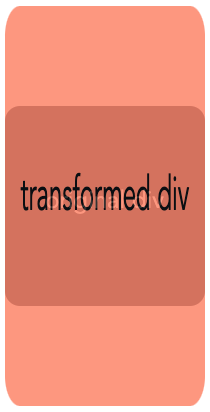### CSS Transform: Rotate

The rotate() function, as you might guess, rotates an element. By default, the element will rotate around its center. We can specify the rotation in terms of degrees, radians, or turns (from 0turn to 1turn):

` `transform: rotate(45deg);``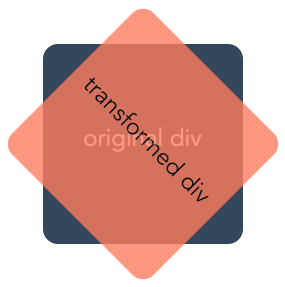` `transform: rotate(0.75turn);``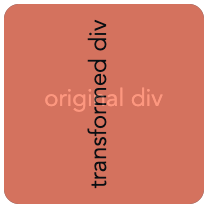### CSS Transform: Skew

The skew() method skews, or slants, an element along its X and/or Y axes. Its arguments specify the horizontal and vertical angle of the skew, respectively.

` `transform: skew(50deg, -15deg);``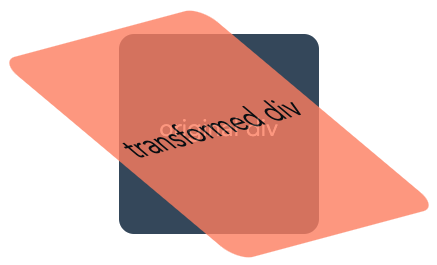skewX() skews our div on the horizontal axis only, and skewY() does the same on the vertical axis only:

` `transform: skewX(50deg);``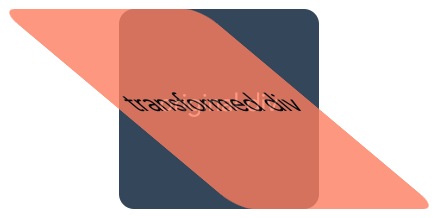` `transform: skewY(50deg);``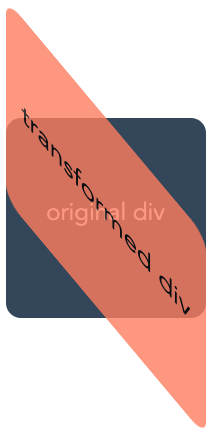### Combining 2D CSS Transformations

If you want to apply multiple transformations to the same CSS selector, you don’t need multiple transform declarations. Instead, you can combine multiple CSS transform values into one declaration by simply listing them with a space in between each one:

` `transform: translate(100px, 20px) scale(0.8) rotate(30deg);``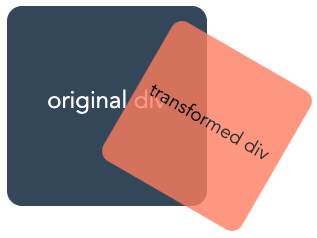## 3D CSS Transformations

Three-dimensional CSS transformations incorporate the Z-axis. If you imagine the width and height of your screen as the X and Y-axes respectively, the Z-axis is the “depth” of your screen.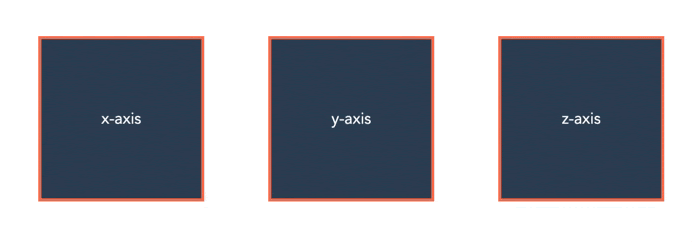Image Source

Here are some ways to transform your elements to add this depth:

### CSS Transform: Perspective

The perspective() value sets the depth of the element on the Z-axis. It toggles how “close” or “far away” the element appears. perspective() is used in conjunction with other 3D transformation methods, as we’ll see next.

### CSS Transform: rotateX() and rotateY()

Like rotate(), the rotateX() and rotateY() values rotate our div, but “around” the X and Y-axes:

` `transform: rotateX(130deg);``` `transform: rotateY(130deg);``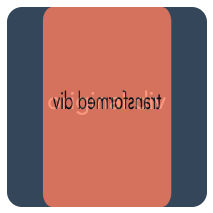When these methods are used with perspective(), the rotated element appears to rotate toward us:

` `/* first image */transform: perspective(500px) rotateY(40deg);/* second image */transform: perspective(500px) rotateY(60deg);/* third image */transform: perspective(500px) rotateY(80deg);``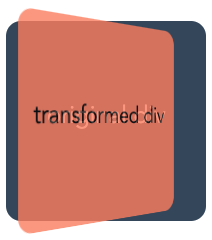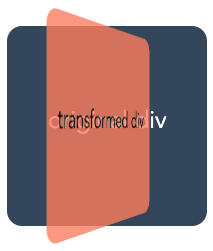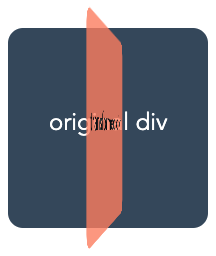### translateZ()

When used with perspective(), the translateZ() creates the effect of an element moving forward or backward on the Z-axis:

` `transform: perspective(500px) translateZ(200px) ;``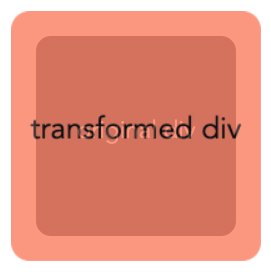## The CSS transform-origin Property

transform-origin is another CSS property that can be used with transform. The transform-origin property changes the position of the origin, the point where the transformation starts or is based around.

This is most clearly demonstrated with the rotate() method: We can use transform-origin to move the center point of rotation:

` `transform: rotate(45deg);transform-origin: top left;``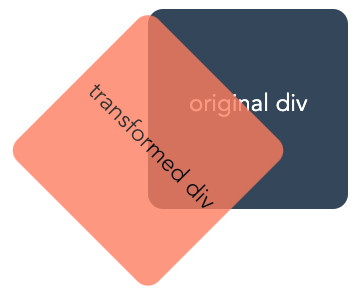` `transform: rotate(45deg);transform-origin: bottom left;``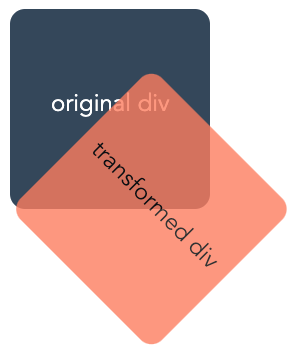` `transform: rotate(45deg);transform-origin: center right;``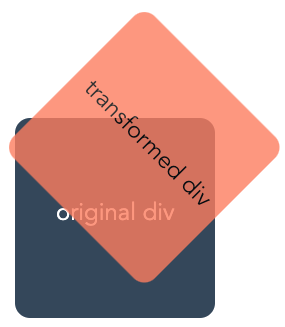The origin can also be specified in pixel distance from the top left corner of the original element:

` `transform: rotate(45deg);transform-origin: 75px 150px;``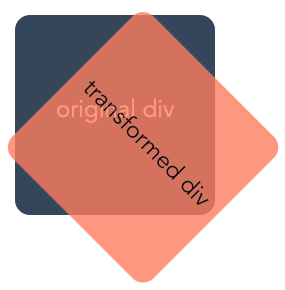## CSS Transform Animations

All of our examples so far have been static...but we can do better than that. Any CSS transform method can be combined with CSS transitions to produce cool CSS animation effects.

For example, we can code an interactive hover button with the scale() method:

` `div {transition: transform 0.5s;}div:hover {transform: scale(1.1);cursor: pointer;}``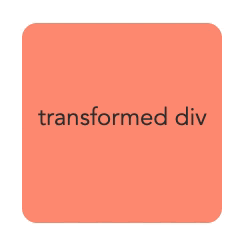...or, produce a pinwheel-like effect with rotate():

` `div {transition: transform 2s;}div:hover {transform: rotate(720deg);}``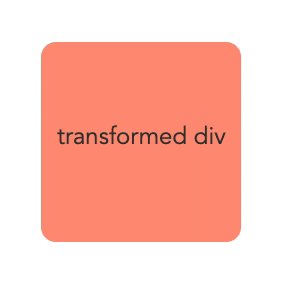## Let’s Review

We’ve covered a lot, so let’s go back over everything we’ve learned:

• transform: A CSS property for changing the shape, position, and orientation of page elements. It can take one or more of the following values:
• translate(): moves an element on the page
• translateX(): moves an element horizontally on the page
• translateY(): moves an element vertically on the page
• translateZ(): moves an element along the Z-axis, “toward” or “away” from the user
• scale(): increases/decreases the size on an element
• scaleX(): increases/decreases the width of an element
• scaleY(): increases/decreases the height of an element
• rotate(): rotates an element (around its center by default)
• rotateX(): rotates an element around the X-axis
• rotateY(): rotates an element around the Y-axis
• skew(): skews an element’s shape
• skewX(): skews an element’s shape horizontally
• skewY(): skews an element’s shape vertically
• perspective(): when used with a 3D transform value, gives the appearance of an element moving toward/away from the user
• transform-origin: A CSS property used with transform to change the origin of the transformation.
• transition: A CSS property to control animation speed. It can be used with transform to animate page elements.

There’s a lot you can do when you combine these powerful techniques — experiment and see what you come up with!

And remember, whatever cool effects you achieve, make sure they’re ultimately serving the user by enhancing the browsing experience, and not by distracting.Originally published Nov 2, 2020 7:00:00 AM, updated December 02 2020

Topics:

Bootstrap & CSS